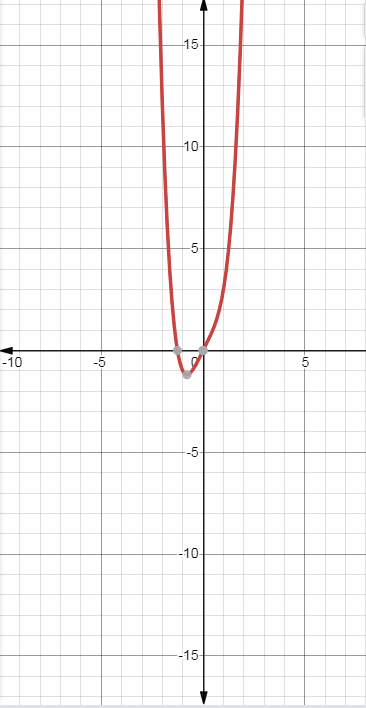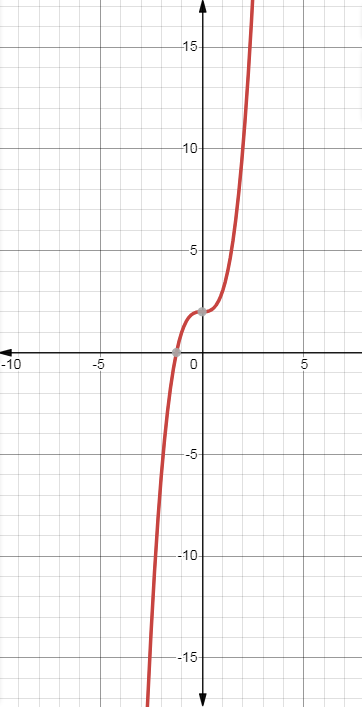# The derivative of the given function by using definition of derivative.### Single Variable Calculus: Concepts...

4th Edition
James Stewart
Publisher: Cengage Learning
ISBN: 9781337687805### Single Variable Calculus: Concepts...

4th Edition
James Stewart
Publisher: Cengage Learning
ISBN: 9781337687805

#### Solutions

Chapter 2.7, Problem 31E

a.

To determine

## To calculate:The derivative of the given function by using definition of derivative.

Expert Solution

Derivative of the function is 2 .

### Explanation of Solution

Given: f(x)=x4+2x

Concept used:

Definition of derivative: f'(x)=limh0f(x+h)f(x)h

f(x)=x4+2x be a given function.

It has to find derivative of the function.

f(x)=x4+2x

f'(x)=limh0f(x+h)f(x)h

f'(x)=limh0(x+h)4+2(x+h)x42xh=limh0h4+4xh3+4hx3+6h2x2+2hh=limh0h3+4xh2+4x3+6hx2+2=2

Hence, derivative of the function is 2 .

b.

To determine

Expert Solution

### Explanation of Solution

Given: f(x)=x4+2x

Concept used:

Definition of derivative: f'(x)=df(x)dx

f(x)=x4+2x be a given function.

It has to find derivative of the function.

f(x)=x4+2x

f'(x)=df(x)dx

f'(x)=d(x4+2x)dx=4x3+2

Now the graph of function and graph of the derivative isRespectively.

### Have a homework question?

Subscribe to bartleby learn! Ask subject matter experts 30 homework questions each month. Plus, you’ll have access to millions of step-by-step textbook answers!